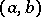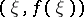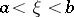# Rolle theorem

(diff) ← Older revision | Latest revision (diff) | Newer revision → (diff)
If a real-valued functionis continuous on some closed interval, has at each interior point of this interval a finite derivative or an infinite derivative of definite sign and at the end points of this interval takes equal values, then in the open intervalthere exists at least one point at which the derivative ofvanishes.
The geometric sense of Rolle's theorem is that on the graph of a functionsatisfying the requirements of the theorem there exists a point,, such that the tangent to the graph at this point is parallel to the-axis.